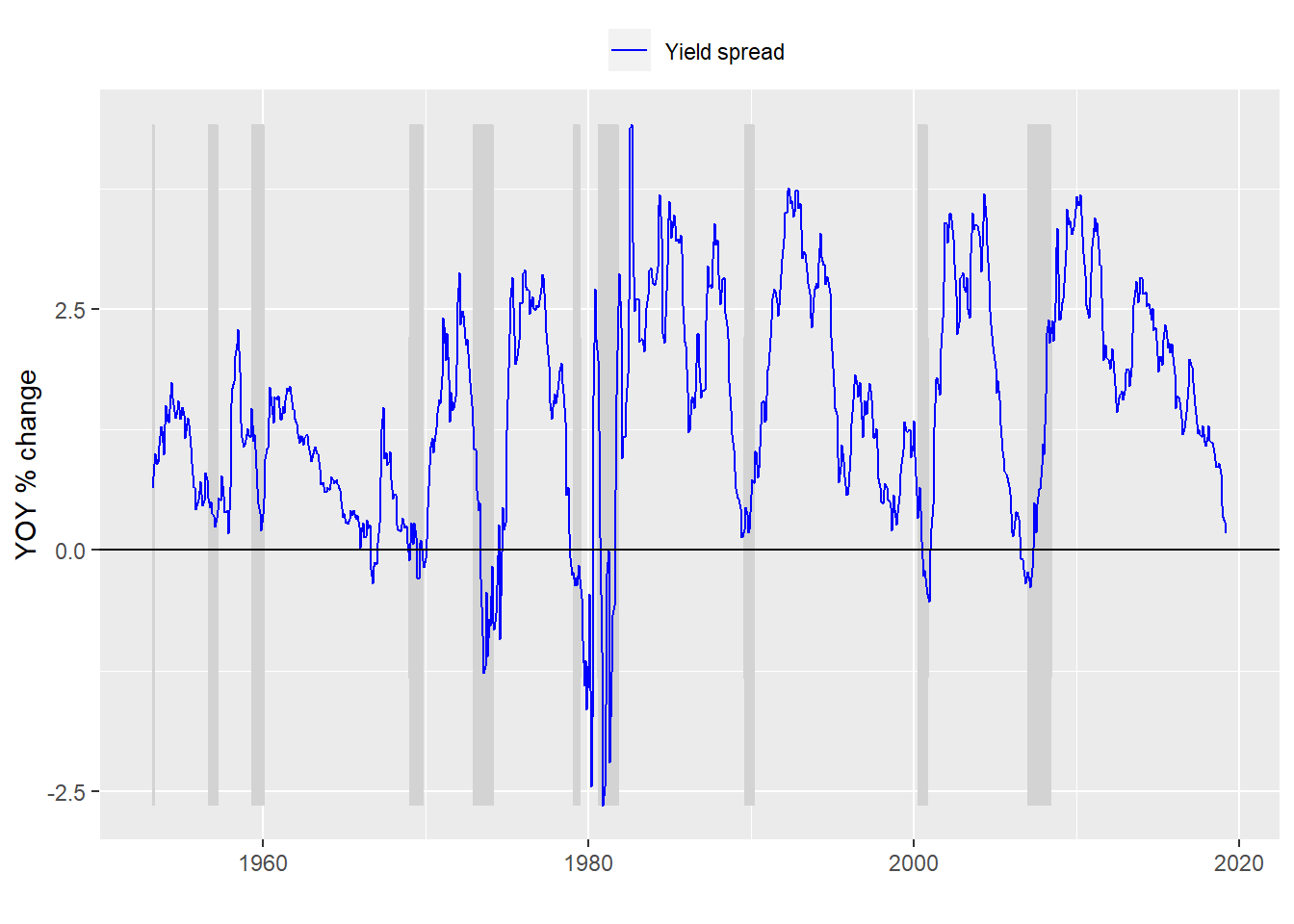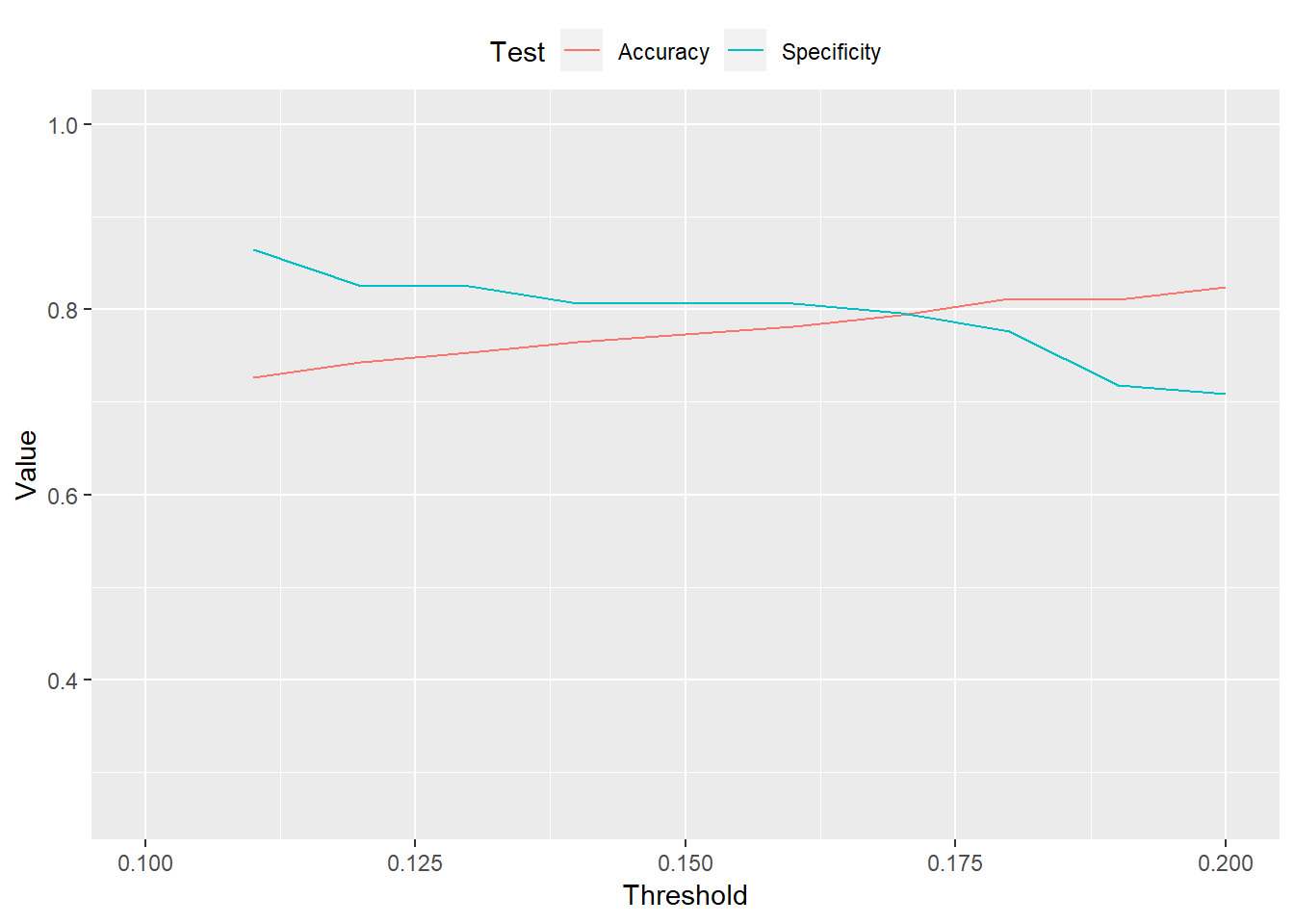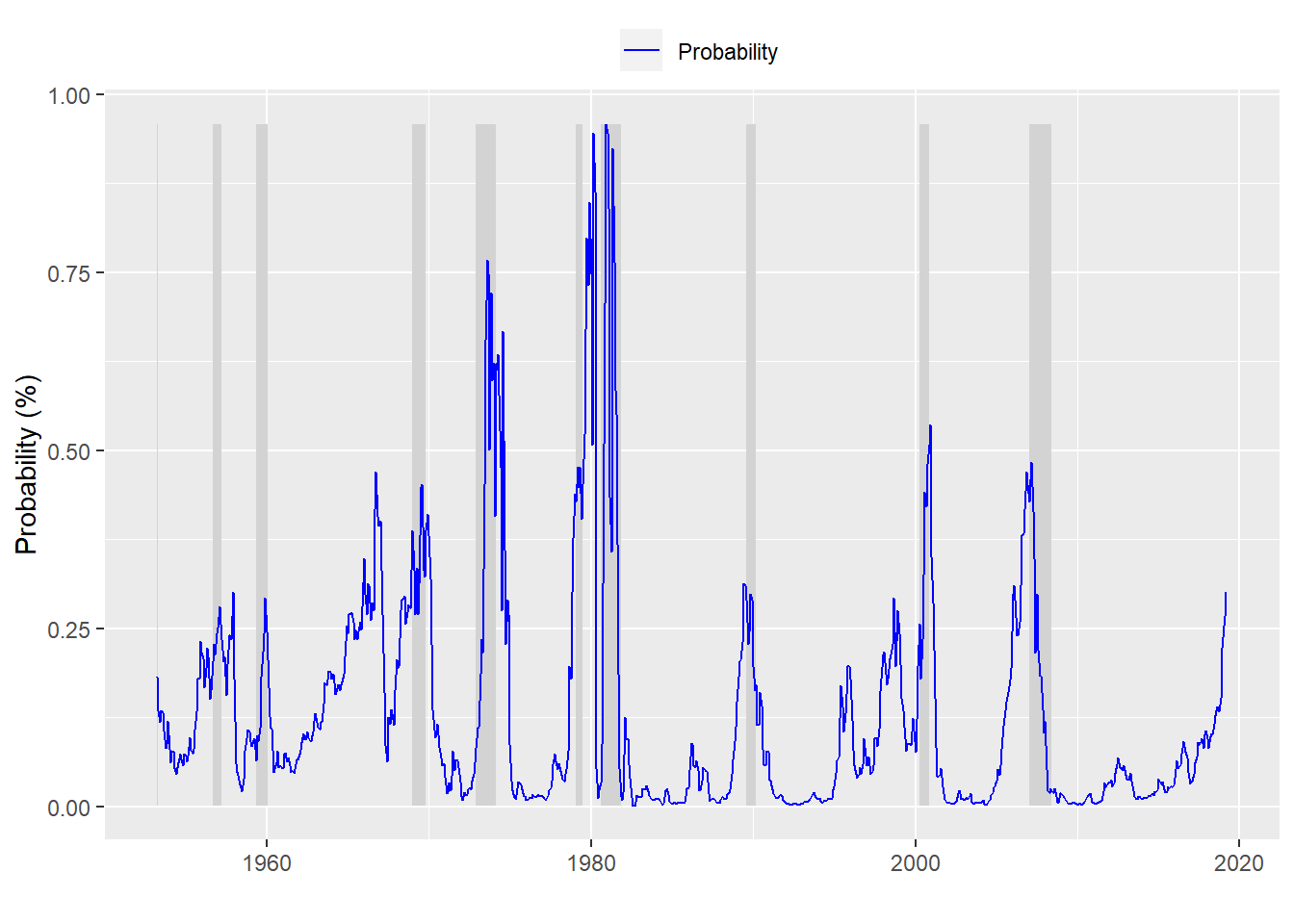# What do machines know about the yield curve?

As we saw in the last post, when we run a model with a 6-month look forward, it does a fairly reasonable job in predicting a recession, assuming we use a threshold closer to recession base rate. In this post, we look at 12-month look forward and then use the best of the two look forward models to test it on out-of-sample data.

## A recession a year from now?

We can dispense with much of the discussion about building the model since we’ve covered that in other posts. Here we’lll just present the data and move on to a machine learning model. Here’s a chart of the yield curve with recessions adjusted for the 12-month look forward.Now we create a model based on that look forward period and present the confusion matrix

Predicted/Actual No recession Recession
No recession 676 86
Recession 13 17

And here are the usual metrics:

With the usual metrics:

• Accuracy: 88%
• Specificity: 17%
• False positive rate: 83%

The model’s accuracy is about the same as the 6-month look forward. While the specification is modestly better. But this comes with the trade-off of a higher false positive rate. But at a very low rate of 2% the trade-off is probably worth it.

Now we look at varying thresholds for the recession predictionAnd for those interested, here is a graph of the probabilities of a recession based on the 12-month look forward. Interestingly, even when the model is in the midst of a recession, it predicts the probability of a recession often less than 50%.This is all well and good (if a little academic). The main problem is that we’ve bulit a model on all the available data. How do we know this model will work on new data? For that we need to use some relatively straightforward machine learning techniques. We’ll split the data into training and test sets. Build the model on the training set and then test its predictive ability on the test set.

We need to consider a couple of issues before we move forward. Where do we split the data? And how reliable should we consider these results since there have only been about 10 recessions in the last 65 years. The second issue is a bit thornier and will shelve that for the next post.

On the first issue, we don’t have a good analytical answer as to where we should split the data. We can run the model on different train/test splits, which we can try in later episodes. But for now we’ll use 1999 as the cut-off.

Predicted/Actual No recession Recession
No recession 216 26
Recession 1 0

The usual metrics:

• Accuracy: 89%
• Specificity: 0%
• False positive rate: 100%

Great…zero ability to predict a recession correctly. But we’re using the 50% threshold as before. If we use a 15% threshold, in line with the recession base rate we generate the following results:

Predicted/Actual No recession Recession
No recession 192 8
Recession 25 18

Revised metrics:

• Accuracy: 86%
• Specificity: 69%
• False positive rate: 31%

This isn’t bad. The accuracy remains high relative to prior models, but specificity increases significantly. Of course, that comes at the cost of a much higher false positive rate. But should we be worried about the effects of such a high false positive rate? That will have to wait.

Here’s the code behind everything above:

# Load package
library(tidyquant)

# Process data
df_12 <- df %>% mutate(usrec = lead(usrec, 12, default = 0))

# Plot data
df_12 %>% ggplot(aes(x = date)) +
scale_colour_manual("",
values = c("blue")) +
ylab("YOY % change") + xlab("") + ylim(c(min(df_12$time_spread), max(df_12$time_spread))) +
geom_hline(yintercept = 0, color = "black") +
theme(legend.position = "top", legend.box.spacing = unit(0.05, "cm"))

# Create model
model_12 <- glm(usrec ~ time_spread, df_12, family = binomial)

# Predict on test set
pred_12 <- predict(model_12, df_12, type = "response")

# Confusion matrix
probs_12 <- rep(0,nrow(df_12))
probs_12[pred_12 > 0.5] <- 1
tab <- table(Predicted = probs_12, Actual = df_12$usrec) rownames(tab) <- c("No recession", "Recession") colnames(tab) <- c("No recession", "Recession") library(printr) tab # Build specification function spec_func <- function(threshold, df){ probs <- pred_12 > threshold tab <- table(probs, df$usrec)
if(nrow(tab) == 1){
return(0)
}else{
return(tab[2,2]/(tab[2,2] + tab[1,2]))
}
}

# Build accuracy function
acc_func <- function(threshold, df){
probs <- pred_12 > threshold
tab <- table(probs, df$usrec) if(nrow(tab) == 1){ return(tab[1,1]/sum(tab)) }else{ return((tab[2,2] + tab[1,1])/sum(tab)) } } # Run specification spec_12 <- c() for(i in 1:20){ spec_12[i] <- spec_func(i*.01, df_12) } # Run accuracy acc_12 <- c() for(i in 1:20){ acc_12[i] <- acc_func(i*.01, df_12) } # Create data frame, tidy, and graph acc_spec_12 <- data.frame(Threshold = seq(0.01, 0.20, 0.01), Accuracy = acc_12, Specificity = spec_12) acc_spec_12 <- acc_spec_12 %>% gather(Test, Value, -Threshold) acc_spec_12 %>% ggplot(aes(Threshold, Value, color = Test)) + geom_line() + xlim(c(0.1,0.2)) + theme(legend.position = "top", legend.box.spacing = unit(0.05, "cm")) # Plot probabilities df_12 %>% mutate(prob = pred_12) %>% ggplot(aes(x = date)) + geom_ribbon(aes(ymin = usrec*min(prob), ymax = usrec*max(prob)), fill = "lightgrey") + geom_line(aes(y = prob, color = "Probability")) + scale_colour_manual("", breaks = c("Probability"), values = c("blue")) + ylab("Probability (%)") + xlab("") + ylim(c(min(pred_12), max(pred_12))) + theme(legend.position = "top", legend.box.spacing = unit(0.05, "cm")) # Create train and test sets start_date_train <- "1953-04-01" end_date_train <- "1998-12-31" train <- df_12 %>% filter(date >= start_date_train, date <= end_date_train) test <- df_12 %>% filter(date > end_date_train) # Fit model on train set train_fit <- glm(usrec ~ time_spread, train, family = binomial) # Predict on test set pred_test <- predict(train_fit, test, type = "response") # Confusion matrix probs_test <- rep(0,nrow(test)) probs_test[pred_test > 0.5] <- 1 tab_test <- table(Predicted = probs_test, Actual = test$usrec)
rownames(tab_test) <- c("No recession", "Recession")
colnames(tab_test) <- c("No recession", "Recession")
tab_test

# Confusion matrix
probs_new <- rep(0,nrow(test))
probs_new[pred_test > 0.15] <- 1
tab_new <- table(Predicted = probs_new, Actual = test\$usrec)
rownames(tab_new) <- c("No recession", "Recession")
colnames(tab_new) <- c("No recession", "Recession")
tab_new